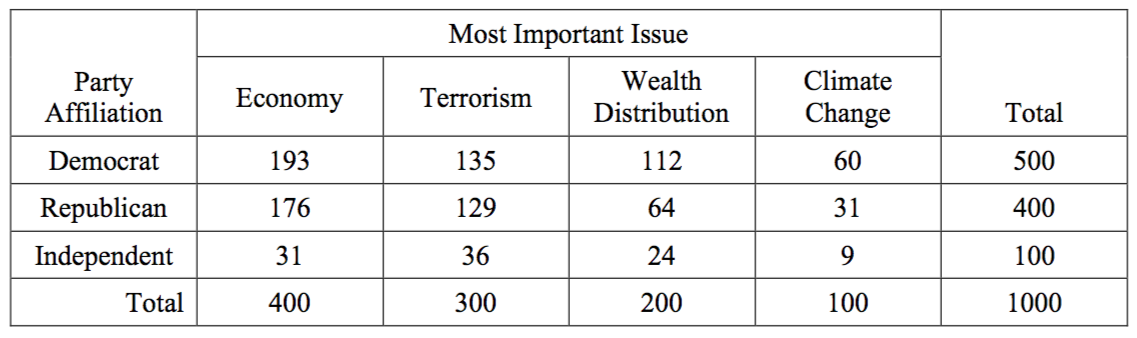# Exercise 1

Professor Professorson, a researcher at Greendale Community College, is interested in the effect of caffeine on the typing speed of students. Professorson obtains a random sample of 8 students who are given 400 mg of caffeine then given a typing test. They type an average of 51.4 words per minute (wpm), with a sample standard deviation of 12.3 wpm. He also obtains a random sample of 13 students who are given a placebo before the typing test. The placebo group types an average of 43.9 wpm, with a sample standard deviation of 15.1 wpm. Assume typing speeds follow a normal distribution in both groups.

(a) Construct a 99% confidence interval for $$\mu_C - \mu_P$$, the true difference in average typing speed between the caffeine and placebo groups. Assume that the two population variances are equal.

(b) Construct a 99% confidence interval for $$\mu_C - \mu_P$$, the true difference in average typing speed between the caffeine and placebo groups. Do not assume that the two population variances are equal. (Use Welch’s T.)

(c) Calculate the the value of the test statistic for testing $$H_0: \ \mu_C = \mu_P$$ versus $$H_1: \mu_C \neq \mu_P$$. Assume that the two population variances are equal.

(d) State the critical value(s) for the test above test, and your statistical decision, using $$\alpha = 0.05$$. Assume that the two population variances are equal.

# Exercise 2

Suppose we randomly sample 400 nucleotides from the human genome. (Consider only a single strand.) Does the occurrence of adenine, thiamine, cytosine and guanine (A, C, T and G) in this sample suggest that nucleotides in the human genome follow a uniform distribution?

Use a $$\chi^2$$ test with a significance level of $$\alpha = 0.01$$ to test the following hypotheses:

• $$H_0: p_A = p_C = p_T = p_G = 0.25$$. ($$p_A$$ is the proportion of adenine in the human genome.)
• $$H_A:$$ At least one of $$p_A, p_C, p_T, p_G$$ is not $$0.25.$$

The data obtained was:

A C T G
Count 90 110 85 115

(a) Calculate the value of the apporpriate test statistic.

(b) State the critical value for this test, and your statistical decision.

Note: Even if A, C, T and G follow a uniform distribution in the human genome, that does not mean that nucleotides occur randomly! Even with a uniform distribution, certain patterns can (and do) occur.

# Exercise 3

At Anytown College, the administration would like the students’ grade distribution to be 25% A’s, 30% B’s, 25% C’s, and 20% D’s. The school’s president thinks that the instructors may not be following this guideline, so he takes a random sample of grades to check his suspicion. A random sample of 30 grades yields 9 A’s, 15 B’s, 3 C’s, and 3 D’s. The president wishes to test

$H_0: \ p_A = 0.25, \ p_B = 0.30, \ p_C = 0.25, \ p_D = 0.20$

(a) Calculate the value of the apporpriate test statistic.

(b) State the critical value for this test, and your statistical decision using a 5% significance level.

# Exercise 4

In a random sample of 1,000 voters in Neverland, each individual was asked to name the issue that is most important to them in the upcoming presidential election. The individuals were also classified by party affiliation. The results were as follows:We wish to test whether political party affiliation and the most important issue are independent.

(a) Calculate the value of the apporpriate test statistic.

(b) Calculate the p-value (approximately) of the apporpriate test statistic, and your statistical decision using a 5% significance level.